Get Free NCERT Solutions for Class 10 Maths Chapter 10 Ex 10.1 PDF. Circles Class 10 Maths NCERT Solutions are extremely helpful while doing your homework. Exercise 10.1 Class 10 Maths NCERT Solutions were prepared by Experienced LearnCBSE.in Teachers. Detailed answers of all the questions in Chapter 10 Maths Class 10 Circles Exercise 10.1 provided in NCERT TextBook.

Topics and Sub Topics in Class 10 Maths Chapter 10 Circles:

 Section Name Topic Name 10 Circles 10.1 Introduction 10.2 Tangent To A Circle 10.3 Number Of Tangents From A Point On A Circle 10.4 Summary

## NCERT Solutions for Class 10 Maths Chapter 10 Circles Ex 10.1

NCERT Solutions for Class 10 Maths Chapter 10 Circles Ex 10.1 are part of NCERT Solutions for Class 10 Maths. Here we have given NCERT Solutions for Class 10 Maths Chapter 10 Circles Ex 10.1

 Board CBSE Textbook NCERT Class Class 10 Subject Maths Chapter Chapter 10 Chapter Name Circles Exercise Ex 10.1 Number of Questions Solved 4 Category NCERT Solutions

Ex 10.1 Class 10 Maths Question 1.
How many tangents can a circle have?
Solution:
There can be infinitely many tangents to a circle.

Ex 10.1 Class 10 Maths Question 2.
Fill in the blanks:
(i) A tangent to a circle intersects it in ………… point(s).
(ii) A line intersecting a circle in two points is called a ………… .
(iii) A circle can have ………………. parallel tangents at the most.
(iv) The common point of a tangent to a circle and the circle is called ……….. .
Solution: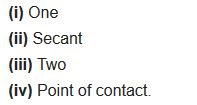You can also download the free PDF of  Ex 10.1 Class 10 Circles NCERT Solutions or save the solution images and take the print out to keep it handy for your exam preparation.

Ex 10.1 Class 10 Maths Question 3.
A tangent PQ at a point P of a circle of radius 5 cm meets a line through the centre O at a point Q so that OQ = 12 cm. Length PQ is
(a) 12 cm
(b) 13 cm
(c) 8.5 cm
(d) $$\sqrt{119}$$ cm
Solution: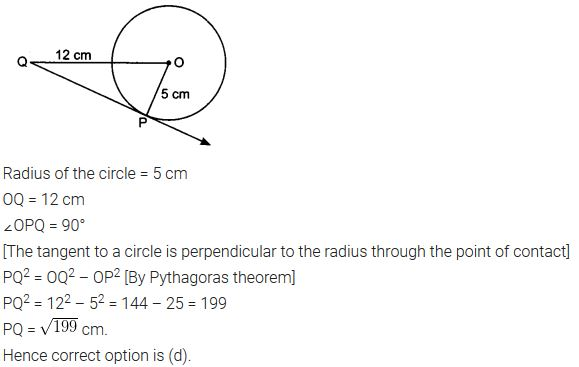Note: PQ = √119

Ex 10.1 Class 10 Maths Question 4.
Draw a circle and two lines parallel to a given line such that one is a tangent and the other, a secant to the circle.
Solution: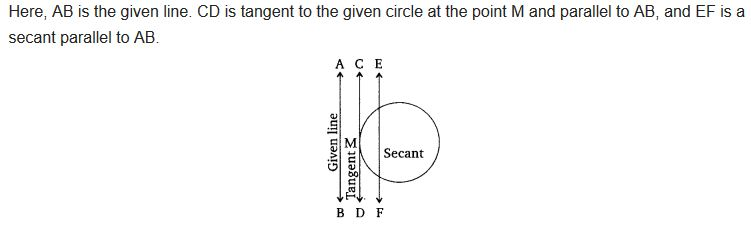### Class 10 Maths Circles Mind Map

#### Introduction

A circle is a set of all points in a plane at a fixed distance from a fixed point in a plane. The fixed point is called the centre of the circle. The fixed distance is called the radius of the circle.

#### Line and a CircleIn Fig. (i), the line PQ and the circle have no common point. In this case, PQ is called a non-intersecting line with respect to the circle. In Fig. (ii), there are two common points A and B that the line PQ and the circle have. In this case, we call the line PQ a secant of the circle. In Fig. (iii), there is only one point A which is common to the line PQ and the circle. In this case, the line is called a tangent to the circle.

#### Tangent

A tangent to a circle is a straight line which touches the circle at only one point. The point where the tangent touches the circle is called point of contact of the tangent to the circle.
A tangent to a circle is a special case of a secant, when the two ends points of its corresponding chord coincides.
Theorem: Tangent at any point on a circle is perpendicular to the radius through the point of contact.CB is the tangent to the given circle touching at A and OA is the radius.
∴ ∠OAB = 90°
(i) At any point on the circle there can be one and only one tangent.
(ii) The line containing the radius through the point of contact is called the normal to the circle at the point.

#### Number of Tangents from a Point to Circle

(i) No tangent can be drawn from the point lying inside the circle, as shown in fig. (i)
(ii) One and only one tangent can be drawn from a point lying on the circle, as shown in fig. (ii)
(iii) Only two tangents can be drawn from an exterior point to a circle, as shown in fig. (iii)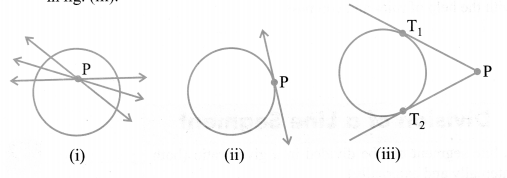#### Length of a Tangent

The length of the segment of a tangent from an external point to the point of contact with the circle is called the length of the tangentIn the given figure, T1 and T2 are the points of contact of the tangents PT1 and PT2 respectively from the external point P.

#### Theorem Related to Length of Tangents From the External Points

The lengths of tangents drawn from an external point to a circle are equal.
i.e.,Here, PQ and PR are the two tangents drawn from P to the circle

### NCERT Solutions for Class 10 Maths Chapter 10 Circles (Hindi Medium) Ex 10.1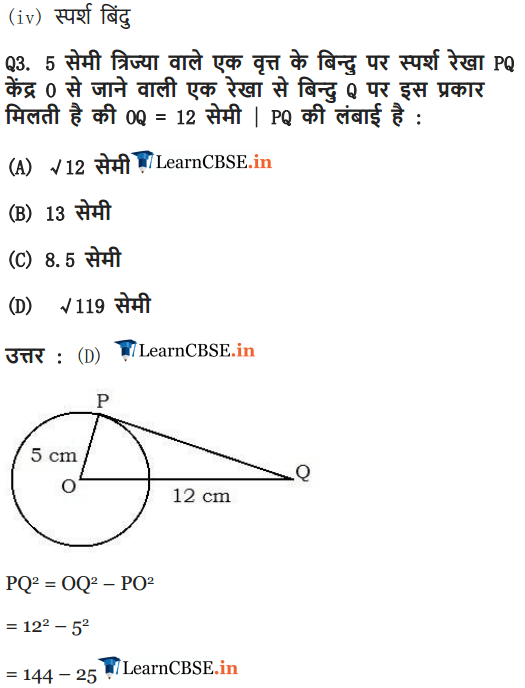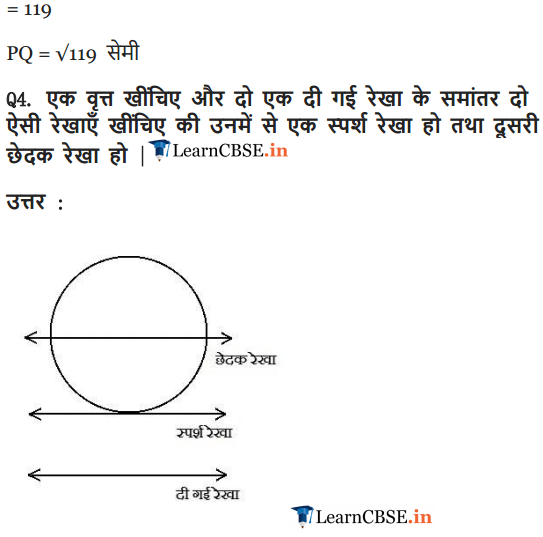More Resources for CBSE Class 10

We hope the NCERT Solutions for Class 10 Maths Chapter 10 Circles Ex 10.1, help you. If you have any query regarding NCERT Solutions for Class 10 Maths Chapter 10 Circles Exercise 10.1, drop a comment below and we will get back to you at the earliest.